• bin -> 无符号十进制>> a = convert_bin2dec('101000001', 0); 一 = 321 bin -> 有符号十进制>> a = convert_bin2dec('101000001', 1); a = -191matlab
• 一个简单的二进制转为十进制，包括有符号位和无符号位的两种转换方式。


C程序之有、无符号位の二进制转十进制
无符号位二进制转换为十进制有符号位二进制转换为十进制关于二进制转十进制说明

为便于理解，不采用自定义函数形式而直接使用主函数main，有需要的自行改写。

无符号位二进制转换为十进制
方式一：
#include <stdio.h>
#include <stdlib.h>
#include <math.h>
#include <string.h>
#define MAXRANGE 256

int main()
{
char binaryScanf[MAXRANGE]; // 无符号字符二进制
int intBinaryScanf[MAXRANGE];   // 存储每个二进制元素
long int decimal = 0;

scanf("%s", binaryScanf);   // 输入二进制数
for(int i = 0, j = strlen(binaryScanf); i < strlen(binaryScanf); i ++)  // 字符二进制逆序转数型
intBinaryScanf[i] = binaryScanf[--j] - '0';	// 字符变数型

for(int i = 0; i < strlen(binaryScanf); i ++)
decimal += intBinaryScanf[i] * (int)pow(2,i);     // 进制二转十公式
printf("%lu\n",decimal);

return 0;
}

方式二：
#include <stdio.h>
#include <stdlib.h>
#include <string.h>
#include <math.h>
int main(void)
{
char str_bin;
gets(str_bin);
int sizeBin = strlen(str_bin);
int sum = 0;
for(int i = 0; i < sizeBin; i ++)
sum += (int)pow(2,(sizeBin-1-i)) * (str_bin[i] - '0');
printf("%d",sum);
return 0;
}

运行结果：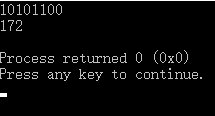有符号位二进制转换为十进制
#include <stdio.h>
#include <stdlib.h>
#include <math.h>
#include <string.h>
#define MAXRANGE 256

int main()
{
char binaryScanf[MAXRANGE]; // 有符号字符二进制
int decimal = 0;   // 所求十进制

scanf("%s", binaryScanf);   // 输入二进制数

if(binaryScanf == '1')   // 符号位 == 1
{
// 逆补码
for(int i = strlen(binaryScanf) - 1; i > 0; i --)
{
binaryScanf[i] -= 1;
if(binaryScanf[i] == '0')
break;
else
binaryScanf[i] = '1';
}
// 逆反码
for(int i = strlen(binaryScanf) - 1; i > 0; i --)
binaryScanf[i] == '0' ? (binaryScanf[i] = '1') : (binaryScanf[i] = '0');
// 二转十公式
for(int j = 0, i = strlen(binaryScanf) - 1; i > 0; i --)
decimal += (binaryScanf[i] - '0') * (int)pow(2,j++);
decimal = 0 - decimal;  // 取负
}
else    // 符号位 == 0
for(int j = 0, i = strlen(binaryScanf) - 1; i > 0; i --)
decimal += (binaryScanf[i] - '0') * (int)pow(2,j++);
printf("%d\n",decimal);
return 0;
}


运行结果：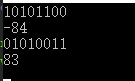符号位 == 0 の处理方法二
int intBinaryScanf[MAXRANGE];   // 存储每个二进制元素

for(int i = 1, j = strlen(binaryScanf) - 1; i < strlen(binaryScanf); i ++)  // 字符二进制逆序转数型
intBinaryScanf[--j] = binaryScanf[i] - '0';	// 字符变数型
for(int i = 0; i < strlen(binaryScanf) - 1; i ++)
decimal += intBinaryScanf[i] * (int)pow(2,i);     // 进制二转十公式

关于二进制转十进制说明

有符号位二转十：

判断符号位（最左端）为0或1若为1：

二进制码 - 1（逆补码）二进制码 反码，即1变0，0变1（逆反码）二进制码公式：除了最左端的符号位，对经前两步处理过的二进制码，自右向左，每个位数依次 × 2 的 0,1,2,3……次方并求和。给求和后的数加个负号“-”。

若为0：

直接套用二进制码公式：除了最左端的符号位，对当前二进制码，自右向左，每个位数依次 × 2 的 0,1,2,3……次方并求和。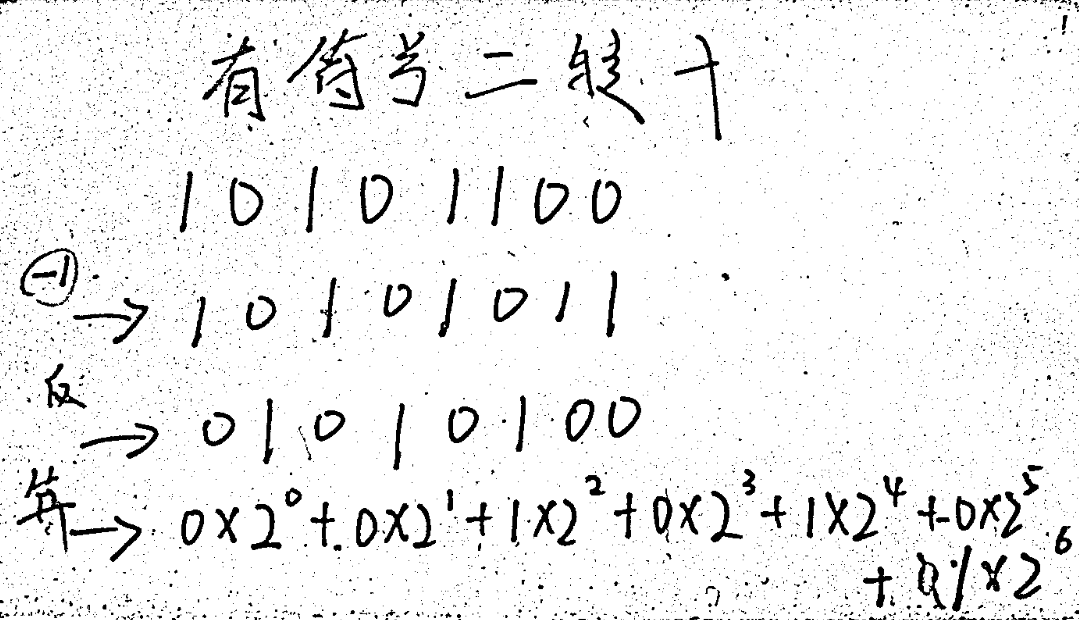无符号位二转十： 直接套用二进制码公式：对当前的二进制码，自右向左，每个位数依次 × 2 的 0,1,2,3……次方并求和。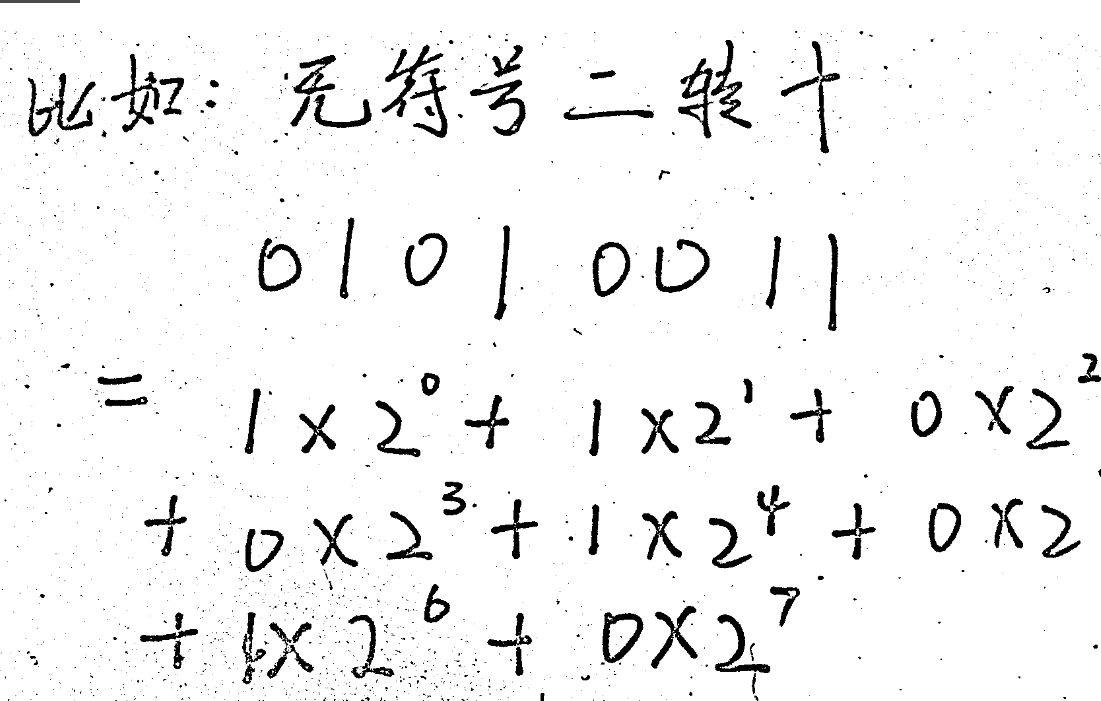展开全文C语言 C 进制转换
• 无符号10进制转换为有符号的十进制，不同于c语言的编写方式。代码matlab
• package day11;... * 输入一个数字n 输出要求的无符号二进制串 * */ public class BinaryNumber { public static void main(String[] args) { Scanner scan = new Scanner(System.in); int[] binary...
package day11;
import java.util.Scanner;
/*
* 输入一个数字n 输出要求的无符号的二进制串
* */
public class BinaryNumber {
public static void main(String[] args) {
Scanner scan = new Scanner(System.in);
int[] binary = new int;
int number = scan.nextInt();
int count = 0;
for (int i = 0; i < binary.length; i++) {
if (number != 0) {
binary[i] = number % 2;
number /= 2;
count++;
}else{
break;
}
}
System.out.println(count);
//逆序输出
for (int i = count-1; i >= 0; i--) {
System.out.print(binary[i]);

}
}
}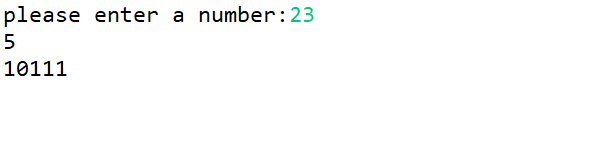展开全文java 算法
•汇编
• % 8 是指变成8位的二进制数，例如 a = 3 ，则 bin 就是 0000_0011 当数字a小于0的时候，这样操作： bin = dec2bin( a + 2^8 , 8 ); % 8 还是8位的意思，比如 a = -3 ,则 bin 就是 1111_1101 操作一个一维数组...
matlab 调用 dec2bin（) 函数，传入负数就报错，做个笔记吧

1.怎么用？
当数字a大于0的时候，直接调用函数
bin = dec2bin( a, 8 );  		% 8 是指变成8位的二进制数，例如 a = 3 ，则 bin 就是 0000_0011

当数字a小于0的时候，这样操作：
bin = dec2bin( a + 2^8 , 8 ); 	% 8 还是8位的意思，比如 a = -3 ,则 bin 就是 1111_1101

操作一个一维数组的时候就可以这样来
% =====  array 是一个一维数组  ===== %

for i = 1 :length(array )
if(array (i) <0)
array (i) = array (i) + 2^8;
end
end

ans = dec2bin(array , 8 ); 		%这个函数 可以直接把数组作为参数输入

例子里面的 8 是指位数，如果需要转化成16位的，就把 所有的 8 换成 16 ！
2.为什么？
dec2bin() 函数会把大于0的整数转换成指定位数的二进制字符串，比如把十进制的 3 转化成 8位的二进制就是：0000_0011 ；小于0的数转化成二进制，应该是转化成对应正整数的补码（ 按位取反 再 + 1），比如3的8位的按位取反就是：1111_1100 ; 为什么要 + 1呢？如果不 + 1，那么 0000_0011 + 1111_1100 就会得到 1111_1111 ,给他加个 1 就变成了 1_0000_0000 ，如果指定了 8 位 ，那么就会直接抛弃第一个1 ，结果就是 0000_0000 ，所以这样 按位取反 再 +1 的操作就是为了对应 3 + （-3） = = 0 这样的计算结果。有了上面的基础，就可以着手准备利用好dec2bin（）函数了；思路就是 把负数 a 的补码（ 绝对值的按位取反 再 + 1），对应的 十进制 的 整数 b 找到，完了dec2bin（b , 8）；有点绕啊，还是举例子，-3 的 补码是 1111_1101，这个字符串对应的十进制就是 253 ; 而 -3 + 2^ 8 == 253;
 power(2,0) + power(2,2)++ power(2,3)+ power(2,4)+ power(2,5)+ power(2,6)+ power(2,7) == 253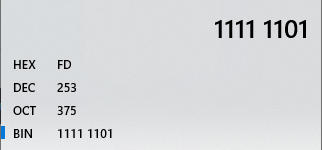结论
正数：直接 dec2bin（）； 负数：先给负数 加一个 2^n ，然后再用 dec2bin（）；
展开全文• //即所有的负值的byte的补码和源码想象为无符号的话之和为256 -(NSString *)unsignedSixteenChangeTenString:(NSString *)sixteenStr { NSString *twoBrand = [self getBinaryByhex:sixteenStr];//二进制有无...

原理就是：正数的源码+负数的源码= 256
比如 补码和源码
//即所有的负值的byte的补码和源码想象为无符号的话之和为256
-(NSString *)unsignedSixteenChangeTenString:(NSString *)sixteenStr
{
NSString *twoBrand = [self getBinaryByhex:sixteenStr];//二进制有无符号 11111111
//    twoBrand = @"10000001";
if (!twoBrand.length) {//防止为空
return @"0";
}
NSString *firstText = [twoBrand substringToIndex:1];

NSString *tenBrand = [self getDecimalSystemWithBinarySystem:[twoBrand substringToIndex:twoBrand.length-1]];

if ([firstText isEqual:@"0"]) {//正数
return tenBrand;
}
tenBrand = [self getDecimalSystemWithBinarySystem:[twoBrand substringToIndex:twoBrand.length]];
NSString *totalBrand = [@"1" stringByAppendingString:[self getNull0TextWithLength:twoBrand.length]];//二进制有无符号 100000000
NSString *totaltenBrand = [self getDecimalSystemWithBinarySystem:totalBrand];
//否则负数
NSInteger value = tenBrand.integerValue-totaltenBrand.integerValue;
return [NSString stringWithFormat:@"%@",@(value)];
}

- (NSString *)getNull0TextWithLength:(NSInteger)length
{
NSString *text = @"";
for (NSInteger i= 0; i<length; i++) {
text = [text stringByAppendingString:@"0"];
}
return text;
}

#pragma mark --- 2进制转10进制
- (NSString *)getDecimalSystemWithBinarySystem:(NSString *)binary{
int ll = 0 ;
int  temp = 0 ;
for (int i = 0; i < binary.length; i ++){
temp = [[binary substringWithRange:NSMakeRange(i, 1)] intValue];
temp = temp * powf(2, binary.length - i - 1);
ll += temp;
}

NSString * result = [NSString stringWithFormat:@"%d",ll];
return result;
}
展开全文ios
• 1.1.二进制转十进制： 函数：bindec(string $binary_string) @param$binary_string 参数表示所要转换的二进制字符串。 @return 返回与\$binary_string参数所表示的二进制数的十进制数等价值。 函数说明： bindec()...
• 编写递归函数void DtoB，实现十进制无符号整数到二进制数的转换。递归提示：例如，如果要输出13的二进制数1101，可以考虑先递归输出13的前面的三个二进制数110，最后再输出结尾的1。 样例输入：13 样例输出：1101 #...c++ c语言 c#
• 帮研究生同学写的一个数制转换代码，将二进制数据转换为十进制数，需转换的数据格式为： 1.每行数据的第一个数为任意数制，仅作为标识，可能为正，也可能为负，无需转换，直接去除； 2.每行余下的数据为二进制数据，...
• 在一些数据处理过程中，需要将一组十进制小数转换成二进制数存储或者计算，这种操作在FPGA的使用中会经常遇到。本文分析了十进制小数转换成二进制数的方法。 1.N位二进制数的取值范围 N位无符号型（unsigned）取值...归一化
• 在计算机编程过程中，各种进制的转换很多，...那么同一组二进制数据在转成有符号和无符号变量时有什么关联呢？ 通常我们将二进制数据进行反码、补码计算，再进行转换，计算比较复杂。 假设一个二进制数据为1000..算法
• c# 带符号8位二进制转十进制方法 /// <summary> /// 有符号二进制10进制 /// </summary> /// <param name="binaryStr"></param> /// <returns></returns> public ...c#
• 思路：处理带小数的二进制转十进制，我们一般可以把它分为两部分，整数部分和小数部分，整数部分二进制转十进制采用逆向累加法，小数二进制转十进制采用正向累加法，然后总体相加即可。 #include&lt;stdio.h&...
• matlab开发-将二进制字符串转换为十进制值。它将二进制流转换为十进制值，每次8位，尽管您可以更改它。
• （左移、右移、无符号右移）的时候发现十进制转二进制数的时候，负数的位数是够的，比如：负整数 -15 的二进制表示是：11111111111111111111111111110001 ，但是 正整数 15 的二进制表示是：1111，抱着好奇心，...
• 把两个无符号的字符，拼接一个有符号的十进制数，方法是：将高位的字符左移8位，然后位或低8位的字符。 （2）判断符号。单独提取最高位的位。方法：要提取的变量位与0x8000 （3）将补码表示的负数，转成...
• 十进制转二进制 方法为：十进制数除2取余法，即十进制数除2，余数为权位上的数，得到的商值继续除依此步骤继续向下运算直到商为0为止。 十进制转换为其他的进制同理 2进制转换为8进制之类的转换可以借助十进制...
• 本文实现的是将十二位的有符号二进制数转换为十进制数： 由于在数字在显示中，如果高位为0会在显示中被省去，所以，这里无法检测到每个数的位数都为12位，也就是无法全部检测到12位的符号位。 比如： 000011110000...matlab
• 目录   一、方法引用 二、代码编写 ... //将n转化为二进制s，s是字符串 int a = Integer.valueOf(s); int b = Integer.parseInt(s); //这是两种将字符串转化为整形的方法，若n是31，结果都为11111...Java
• 1023~+1023的数据转换为二进制，其实这个问题完全可以通过Matlab编程来解决，但是呢，得到的结果要重新复制进Excel表格中，也没有那么方便，所以想直接用Excel中的公式来进行十进制转二进制操作。   Excel中自带...Excel DEC2BIN
• #include <iostream> #include <algorithm> #include <cstring> #include <fstream> using namespace std; string toNumber(int n){ string s; if(n==0) ... while(n)...
• 点分十进制（Dotted Decimal Notation）全称为点分（点式）十进制表示法， 是IPv4的IP地址标识方法。 IPv4中用四个字节表示一个IP地址，每个字节按照十进制表示为0~255。 点分十进制就是用4个从0~255的数字，来表示...
• 输入1个无符号二进制数串，编写程序将其转换对应的十进制数，并输出。 输入格式: 输入1个二进制数串（至少1位且不超过16位）。 输出格式: 输出转换后的十进制数。 输入样例: 0110110001100 输出样例: 3468 源...c语言
• 二进制，八进制，十进制，十六进制之间的关系是什么?浮点数是什么回事？ 本文内容参考自王达老师的《深入理解计算机网络》一书&amp;amp;amp;amp;amp;amp;amp;amp;amp;lt;中国水利水电出版社&amp;amp;amp;amp...
• 最近在用Python写遗传算法时，发现需要将十进制的整数转换成二进制数，那么怎么来转换呢？当然如果你学过进制转换的有关计算方法，你可以手动编写一些函数来实现，不过总体来说还是比较麻烦的，这里介绍Python内置的...Python 小技巧 基础
• dec2bin()：该函数实现从十进制二进制的转换。调用格式：b=dec2bin(a)。其中b为二进制，a为十进制。 bin2dec()：该函数实现从二进制十进制的转换，调用格式：a=bin2dec(b)。其中b为二进制，a为十进制。参数b的...matlab
• 摘自https://baike.baidu.com/item/%E5%8D%81%E8%BF%9B%E5%88%B6%E8%BD%AC%E4%BA%8C%E8%BF%9B%E5%88%B6 python实现进制转换：... 十进制转二进制 编辑 1. 十进制整数转换为二进制整数 十进制整数...
• 1. 从键盘输入某个十进制整数数，转换对应的二进制整数并输出。 public static void main(String[] args) { Demo3 d2=new Demo3(); d2.arithmetic1(); d2.arithmetic2(); } private void arithmetic1(){ //...
• 从键盘输入1个小于65536的无符号十进制整数，将其转换16位二进制，并输出。 具体要求完成的功能如下： 1）如输入负数，则输出 “数据小于0” 2）如超过65535，则输出“超过最大数” 3）输出转换过的16位二进制数...c语言...